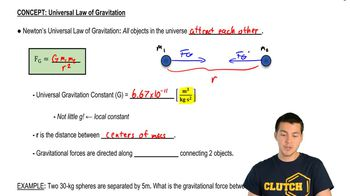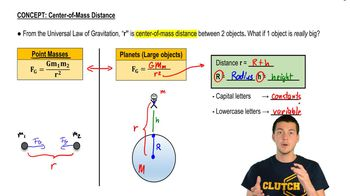Start typing, then use the up and down arrows to select an option from the list.
4:54 minutes
Problem 13a
Textbook Question

# The point masses m and 2m lie along the x-axis, with m at the origin and 2m at x = L. A third point mass M is moved along the x-axis. (a) At what point is the net gravitational force on M due to the other two masses equal to zero?Verified Solution
This video solution was recommended by our tutors as helpful for the problem above.
49views